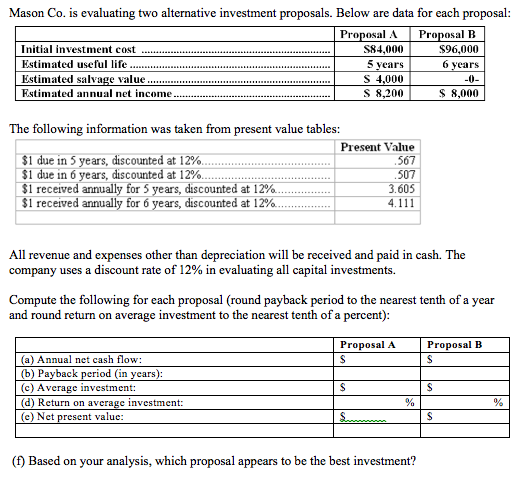# Question & Answer: Mason Co. is evaluating two alternative investment proposals. Below are data for each proposal: The following i…..

Please see attached picture of the problem which needs to be solved.

Thanks!

Don't use plagiarized sources. Get Your Custom Essay on
Question & Answer: Mason Co. is evaluating two alternative investment proposals. Below are data for each proposal: The following i…..
GET AN ESSAY WRITTEN FOR YOU FROM AS LOW AS \$13/PAGEMason Co. is evaluating two alternative investment proposals. Below are data for each proposal: The following information was taken from present value tables: All revenue and expenses other than depreciation will be received and paid in cash. The company uses a discount rate of 12% in evaluating all capital investments. Compute the following for each proposal (round payback period to the nearest tenth of a year and round return on average investment to the nearest tenth of a percent): (f) Based on your analysis, which proposal appears to be the best investment?

a)Annual net cash flow:

Annual net cash flow for Proposal A=(Net Income) +Depreciation (non cash expense)

Depreciation =(Initial investment-Salvage value)/useful life

Depreciation=(84000-4000)/5=\$16,000

Annual net cash flow for Proposal A=(8200+16000)=\$24,200

Annual net cash flow for Proposal B=(Net Income) +Depreciation (non cash expense)

Depreciation =(Initial investment-Salvage value)/useful life

Depreciation=(96000-0)/6=\$16,000

Annual net cash flow of Proposal B=(8000+16000)=\$24,000

b)Pay back period

Pay back period is the period when the initial investment is recovered

Payback period of proposal A=84000/24200=3.47 years

Payback period of proposal b=96000/24000=4 years

c)Average Investment:

Average investment=(Initial investment +Scrap value)/2

Average investment Proposal A=(84000+4000)/2=\$44,000

Average investment Proposal B=(96000+0)/2=\$48,000

d)Return on average investment:

Return on average investment =Net annual income/Average investment

Return on average investment of Proposal A=8200/44000=0.1864=18.64%

Return on average investment of Proposal B=8000/48000=0.1667=16.67%

e)Net Present value:

Net Present value(NPV) of Proposal A;

Present Value (PV) of Salvage value=4000*0.567=\$2,268

PV of annual net cash flow=24200*3.605=\$87,241

Net Present value(NPV) of Proposal A=2268+87241-84000=\$5,509

Net Present value(NPV) of Proposal B;

Present Value (PV) of Salvage value=0

PV of annual net cash flow=24000*4.111=\$98,664

Net Present value(NPV) of Proposal B=0+98664-96000=\$2,664

 Proposal A Proposal B a) Annual net cash flow \$24,200 \$24,000 b) Pay back period in years 3.47 4.00 c) Average Investment \$44,000 \$48,000 d) Return on average investment 18.64% 16.67% e) Net Present Value(NPV) \$5,509 \$2,664

(f) Based on the above analysis Proposal A appears to be best , because:

• Higher NPV
• Lower investment
• Higher return on average investment
• Lower pay back period